At timescales in the range of pico - nano seconds, the magnetic response is determined by the characteristic frequency of the magnetization precession and corresponds to typical ferromagnetic resonance experiment (FMR) [Slichter 89], where the small external perturbation is applied to the systems with the frequency typically of the orderto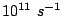. In the theory of FMR the dynamic Landau-Lifshitz-Gilbert (LLG) equation is linearized and the solution is being sought as a linear dynamic response to the small perturbation in terms of the trigonometric functions proportional to that of the external excitation. However, at this timescale the system is in a dynamical regime, and if the external perturbation is not small, it possesses many characteristics inherent to the dynamics of nonlinear systems.

Typical magnetometer measurements of the magnetization response to external fields are on the opposite end of the timescale range. At this scale, the frequency of excitation is much smaller than that of precession frequency or the typical inverse relaxation time, determined by the damping parameter. From the computational point of view, the corresponding response could be calculated in the stationary approximation, i.e. minimizing the total magnetic energy at each external magnetic field value. The intermediate timescale corresponds to that of the adiabatic dynamics in which the dynamical information could be taken on average. In this regime the precession time is faster than the external perturbation, but the relaxation is not fast enough to consider the system as stationary at each point. An example of such dynamics is illustrated in Fig. 2.11. One of the attempts to describe the adiabatic dynamics of single domain particles is due to G. Bertotti et al. [Bertotti 04], who calculated a slow magnetic energy variation in the presence of constant applied field.We study the motion of a single domain particle under an adiabatically varying magnetic field in the small amplitude approximation. The system is the same described in the previous section, but in this case the position of the minimum will also slowly change with time and the equations,(2.50)

determine the coordinates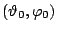of the stationary points, including minima. The number of minima is two if the applied field is less than the critical field determined by the Stoner-Wohlfarth astroid [Stoner 48] and only one if it does not.

In this case the amplitude of precessionis no more constant. It can be found from the Eqs. (2.44) and (2.45) in a linear approximation and the supposition that the applied field is a slow varying function of time, i.ewith. Consequently,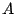andare also slowly varying functions of time. The solution for the magnetic moment motion is then: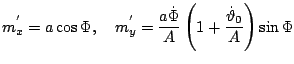(2.51)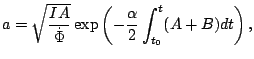(2.52)whereis a constant dependent of the initial conditions. The quantityis the square of the instantaneous frequency of rotations and its first term,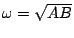, is the ferromagnetic resonance frequency, the second term represents the renormalization by the friction. The third term is a geometrical Berry-Hannay [Berry 85] phase that depends on the trajectory. In order to know the current phase, we need to know the trajectory in the space of parameters and even in the case of equal initial and final applied fields (case that includes a periodic applied field) there will be an additional phase in the moment motion.

The adiabaticity condition that the variation of the field has to be less than the ferromagnetic resonance frequency can be rewritten as. However, the variable magnetic field contains two components in the plane and can be dependent on two adiabatic parameters having different rates of variation. Therefore, the former inequality must be complemented by the additional conditions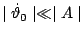,.

2008-04-04